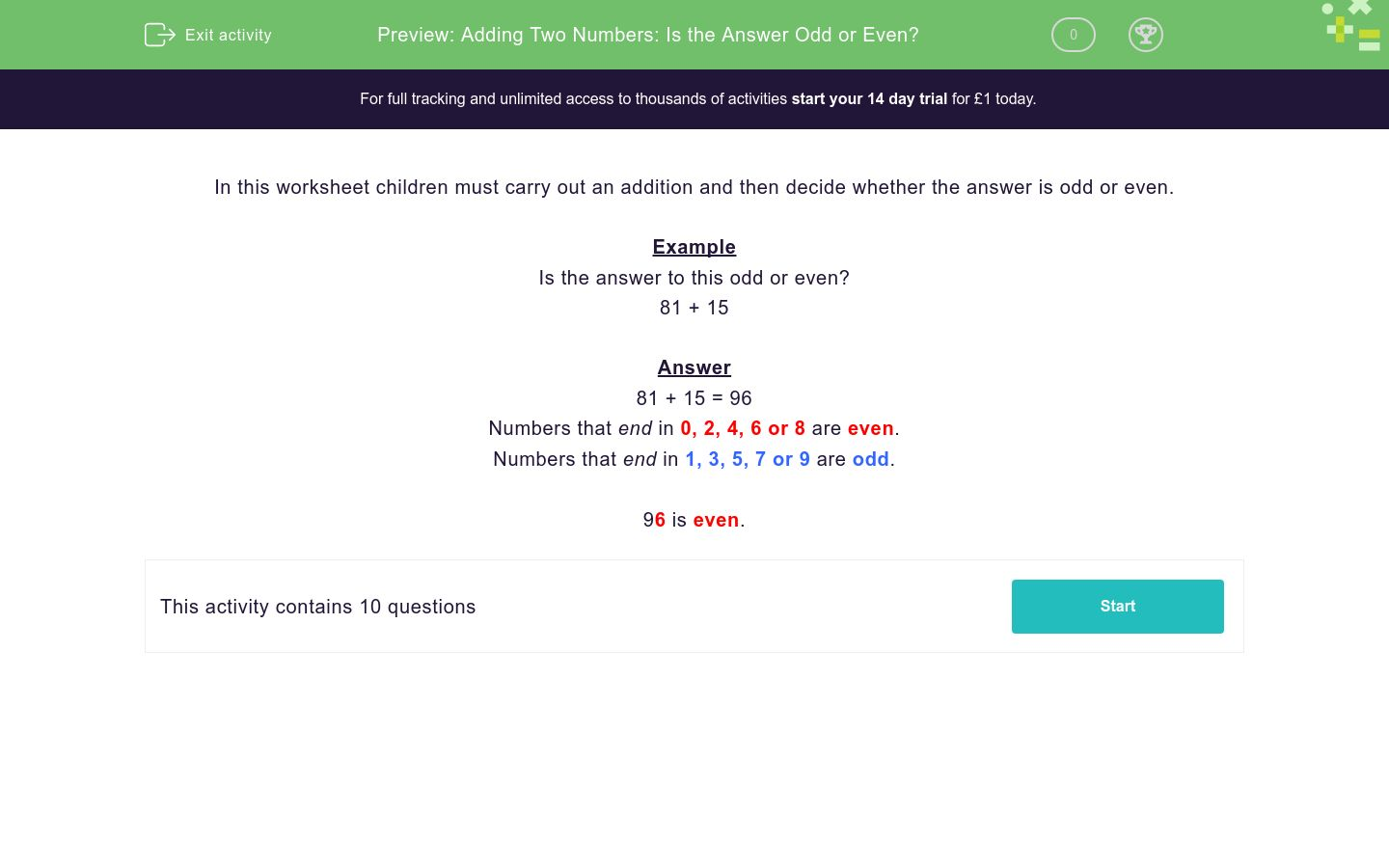### Comprehensive & curriculum aligned

In this worksheet, students carry out an addition of numbers with up to three digits and decide whether the sum is odd or even.Key stage:  KS 2

Curriculum topic:   Number: Addition and Subtraction

Curriculum subtopic:   Use Written Addition/Subtraction to Three Digits

Difficulty level:### QUESTION 1 of 10

In this worksheet children must carry out an addition and then decide whether the answer is odd or even.

Example

Is the answer to this odd or even?

81 + 15

81 + 15 = 96

Numbers that end in 0, 2, 4, 6 or 8 are even.

Numbers that end in 1, 3, 5, 7 or 9 are odd.

96 is even.

---- OR ----